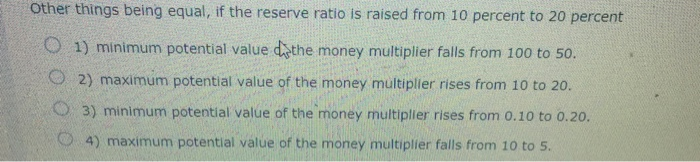### Create an Account

Home / Questions / Other things being equal, if the reserve ratio is raised from 10 percent to 20 percent min...

# Other things being equal, if the reserve ratio is raised from 10 percent to 20 percent minimum potential value of the money multiplier falls from 100 to 50. maximum potential value of the money

Other things being equal, if the reserve ratio is raised from 10 percent to 20 percent minimum potential value of the money multiplier falls from 100 to 50. maximum potential value of the money multiplier rises from 10 to 20. minimum potential value of the money multiplier rises from 0.10 to 0.20. maximum potential value of the money multiplier falls from 10 to 5.Jul 05 2021 View more View LessSubscribe To Get Solution